Reach Us+44-1522-440391
Meta-Analysis of Quantitative Trait Association and Mapping Studies using Parametric and Non-Parametric Models | OMICS International
Journal of Biometrics & Biostatistics

Like us on:

All submissions of the EM system will be redirected to Online Manuscript Submission System. Authors are requested to submit articles directly to Online Manuscript Submission System of respective journal.

Meta-Analysis of Quantitative Trait Association and Mapping Studies using Parametric and Non-Parametric Models

Xiao-Lin Wu1*, Daniel Gianola1,2, Zhi-Liang Hu3 and James M. Reecy3

1Department of Dairy Science, Department of Animal Sciences, University of Wisconsin, Madison, WI 53719, USA

2Department of Biostatistics and Medical Bioinformatics, University of Wisconsin, Madison, WI 53706, USA

3Department of Animal Science, Center for Integrated Animal Genomics, Iowa State University, Ames, IA 50011-3150, USA

*Corresponding Author:
Xiao-Lin Wu
1675 Observatory Docter
Department of Dairy Science
University of Wisconsin
Tel: (608) 263-7824
Fax: (608) 263-9412
E-mail: [email protected]

Received date: June 15, 2011; Accepted date: July 14, 2011; Published date: October 30, 2011

Citation: Wu XL, Gianola D, Hu ZL, Reecy JM (2011) Meta-Analysis of Quantitative Trait Association and Mapping Studies using Parametric and Non-Parametric Models. J Biomet Biostat S1:001 doi:10.4172/2155-6180.S1-001

Copyright: © 2011 Wu XL, et al. This is an open-access article distributed under the terms of the Creative Commons Attribution License, which permits unrestricted use, distribution, and reproduction in any medium, provided the original author and source are credited.

Visit for more related articles at Journal of Biometrics & Biostatistics

Abstract

Meta-analysis is an important method for integration of information from multiple studies. In quantitative trait association and mapping experiments, combining results from several studies allows greater statistical power for detection of causal loci and more precise estimation of their effects, and thus can yield stronger conclusions than individual studies. Various meta-analysis methods have been proposed for synthesizing information from multiple candidate gene studies and QTL mapping experiments, but there are several questions and challenges associated with these methods. For example, meta-analytic fixed-effect models assume homogeneity of outcomes from individual studies, which may not always be true. Whereas random-effect models takes into account the heterogeneity among studies they typically assume a normal distribution of study-specific outcomes. However in reality, the observed distribution pattern tends to be multi-modal, suggesting a mixture whose underlying components are not directly observable. In this paper, we examine several existing parametric meta-analysis methods, and propose the use of a non-parametric model with a Dirichlet process prior (DPP), which relaxes the normality assumptions about study- specific outcomes. With a DPP model, the posterior distribution of outcomes is discrete, reflecting a clustering property that may have biological implications. Features of these methods were illustrated and compared using both simulation data and real QTL data extracted from the Animal QTLdb (http://www.animalgenome.org/cgi-bin/QTLdb/index). The meta analysis of reported average daily body weight gain (ADG) QTL suggested that there could be from six to eight distinct ADG QTL on swine chromosome 1

Keywords

Average daily gain; Dirichlet process prior; Meta-analysis; Markov chain Monte Carlo; Non-parametric models; Quantitative trait loci (QTL); Swine

Introduction

Many quantitative trait association and mapping studies have been conducted in animals and plants in the past 20 years. Although these studies were conducted independently, they could address the same or similar subjects or questions. Thus, their results can be combined to refine conclusions drawn from these studies. Often, we may ask how a causative locus identified for a given trait in one population corresponds to those detected in other populations. Or, does a detected QTL show consistent effect in several populations? While individual studies may be different in reference populations, experimental designs, and many other respects, a well-conducted meta-analysis can provide stronger appraisal of available evidence, and hence reducing uncertainty and disagreement among studies [1-3].

The term, meta-analysis, was coined by G. V. Glass in 1976 when he proposed that distinct methods are needed to integrate the findings from a body of research . Since then, meta-analysis has become an important method for quantitative aggregation and synthesis of knowledge from independent studies in medical, social, and behavioral sciences  as well as in studies of candidate genes and QTLs [6-9] Broadly speaking, a meta-analysis is a quantitative review and synthesis of the results obtained from related but independent studies using appropriate statistical methods . In QTL studies, for example, pooling of results across several studies provides greater statistical power for QTL detection and more precise estimation of their effects, leading to conclusions that are stronger relative to individual studies . The recent development of QTL databases, such as Animal QTLdb [12-14] has provided platforms with which QTL results from individual studies can be compared, combined and synthesized.

However, combining QTL results across several studies can be very challenging because they differ in many aspects. For examples, studies may use different marker densities, linkage disequilibrium, sample sizes, population types, experimental designs, and statistical methods. Hence, heterogeneity may be extensive and difference may be due to between-study variation of true effect sizes as well as chance variations resulting from sampling of individuals into studies. Roughly speaking, two categories of meta-analysis methods have been proposed for the analysis of candidate gene and QTL research. A meta-analytic fixed-effect model assumes homogeneity of outcomes from individual studies, which may not be true in practice. Whereas a meta-analytic random-effect model takes account of heterogeneity among studies, it typically assume a normal distribution of study-specific outcomes. However, such a distribution may be multi-modal . In reality, population stratification and admixture are common in practice, leading to a mixture with unobservable underlying components .

In this paper, we discuss several parametric methods applicable to meta-analysis of quantitative trait association and mapping studies, and propose the use of a non-parametric Bayesian model with a Dirichlet process prior (referred to as the DPP model) for QTL meta-analysis. The DPP model relaxes the normality assumption of study-specific effects by replacing it with a more general, discrete, “nonparametric prior”. It also handles mixture data with an unknown number of components. Features of these models were illustrated and compared using simulation data and real QTL mapping data extracted from the Animal QTLdb.

Statistical Methods

Parametric models for meta-QTL analysis

Consider an outcome, say effect size of a candidate gene (or a putative QTL). Assume that we plan to combine results from k primary studies. For individual study i, let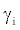be a point estimate of the true gene effect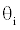, such that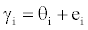(1)

where ei is a residual term. If the study sample sizes are moderate or large, eachis approximately normally distributed according to the central limit theorem. That is,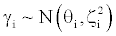, for i =1,...,k , (2)

where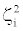is the variance ofThe quantityis typically assumed to be known in a meta-analysis and approximated by the sample variance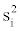The analysis under homogeneity assumes that the unknown parameter is the same over all independent studies,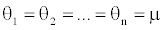, and the only source of uncertainty comes from the sampling of individuals into studies. Then, the maximum likelihood (ML) estimation of μ is given by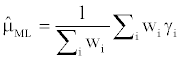with standard error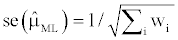, (3)

where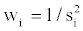The ML estimate of μ is the weighted average of the estimates of the effects in the k studies, with weights equal to. This is the basis for the traditional fixed-effect meta-analysis. Use of inverse variance weights minimizes the variance of estimator of parameter μ. If all weights are equal (i.e.,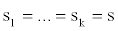) ,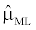becomes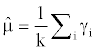with standard error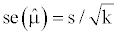. (4)

A statistical test of homogeneity uses the statistic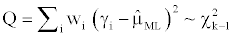(5)

where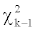is a Chi-squared random variable with k-1 degrees of freedom. If Q is not greater than the 100(1−α) percentile of thedistribution, then the null hypothesis holds and we would conclude that the k studies share a common mean μ (which is estimated by). Otherwise, the alternative hypothesis Ha is accepted, and we would proceed either by attempting to identifying sources of heterogeneity in the population or by fitting a meta-analytic random-effects model instead.

Meta-analytic random-effects model

In real situations, heterogeneity is present and should be considered in the analysis. In a meta-analysis of QTL mapping experiments, for example, two types of heterogeneity are of primary interest : locus and effect size heterogeneity. Under the scenario of locus heterogeneity, a locus could affect the trait of interest in one population but it might have no effect in another one. Likewise, the same locus may influence the trait in all populations, but its effect size may vary over populations. The latter is referred to as size heterogeneity, which happens, for example, when the frequency of the causal allele is much smaller in some populations than in others or when lcoi interact, or when there are differences in environmental variability. In the presence of locus or size heterogeneity, differences among studies arise from both betweenstudy variation of true effect size and chance variation due to sampling of individuals within studies. Thus, a fixed-effects model tends to underestimate variability and generate p-values which are too low. If between-study variation is not accounted for properly, meta-analytic results will overstate significance.

A heterogeneity model assumes that the true study-specific effects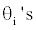, for i =1,...,k , are different from each other and vary according to some distribution, e.g., a normal distribution with mean μ and variance σ2. That is,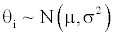. (6)

Then,can be expressed linearly as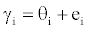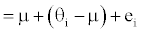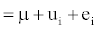, (7)

where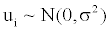. This is a meta-analytic random-effects model. Marginally, the distribution ofis approximated as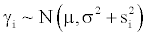(8)

If σ2 = 0 , model (8) reduces to the meta-analytic fixed-effect model.

Assuming σ2 is known, the ML estimate of μ can be obtained similarly as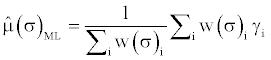(9)

where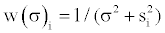. Thus, the estimator of μ is also a weighted average, but the weight is adjusted to take into account the additional variability between studies (σ2 ). The restricted maximum likelihood (REML) approach can be used to estimate the variance components in the model. The REML estimate of σ2 can be obtained by iterating with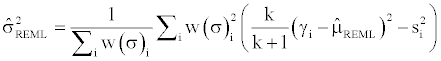, (10)

where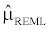is calculated using the same formula as that of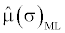but replacing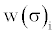with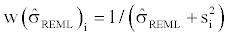.

Bayesian implementation

Within the Bayesian framework, all unknowns are treated as random. Under the homogeneity assumption (which corresponds to the meta-analytical fixed-effect model), a normal prior distribution,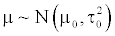, is assigned to μ, where μ0 andτ02 are known hyperparameters. The Bayesian analysis incorporates information from both the prior and the data to obtain posterior inference about the unknown parameter. It can be shown that the conditional posterior distribution of μ is also normal.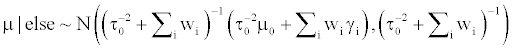, (11)

where “else” represents the data, all parameters other than μ, and all known hyper-parameters. Hence, the posterior mean of μ is: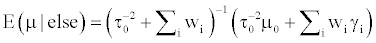. (12)

If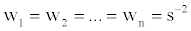, then we have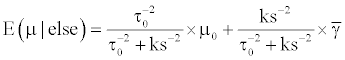(13)

where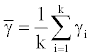. Hence, the posterior mean of μ is the weighted average of the prior mean (μ0) and of the data mean (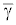), and the weights are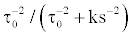and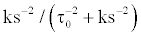, respectively. If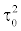is very large (which approximates a flat prior distribution for μ), then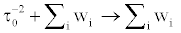and the posterior mean of μ in (12) coincides with the maximum likelihood estimate in (3).

Under the heterogeneity assumption (i.e., random-effects model), if σ 2 is known and μ follows a normal prior distribution a priori, that is,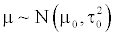, the posterior mean of μ takes a form similar to (12):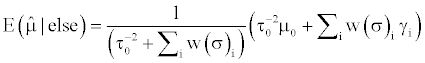, (14)

However, σ2 is unknown. In a Bayesian model, a scaled inverse chi-square prior distribution is typically assumed to σ2 :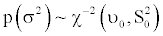, where υ0 andS02 are the (known) degrees of freedom and scale parameters, respectively. Then, draws from the posterior distributions of σ2 , μ and u's can be generated using a Gibbs sampler, by iteratively simulating values from their respective fully conditional distributions: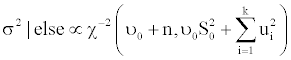(15)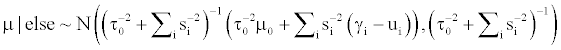(16)

and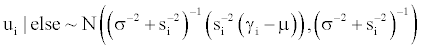for i =1,...,k . (17)

Bayesian non-parametric model with a Dirichlet process prior

Recall that θi =μ + ui and ui ~ N (0,σ2 ) in the parametric randomeffects model. However, the normality assumption, ui ~ N (0,σ2 ) , lacks neither theoretical nor empirical support. Hence, the DPP model can be used instead, because it makes a weaker assumption about the distribution of study-specific outcomes.

In the DPP model, we replace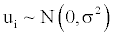with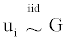, ∀i, where G is some general distribution, G ~π , and π is a “nonparametric prior”. A choice for π is the Dirichlet process [18,19]. That is,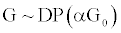(18)

Here, G0 is a “baseline” distribution function, which is also referred to as the “center” of the DP prior in the sense that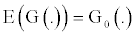, and α is interpreted as a precision parameter indicating the degree of concentration of the prior on G around some parametric family. In particular, we assume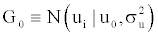, where u0 can take a fixed value (say u0 = 0 ) or it can be treated as an unknown quantity in the model. In the latter case, a prior distribution about u0 is needed in the Bayesian model. To complete the model specification, independent hyper-priors are assumed as follows: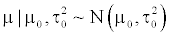, (19)

and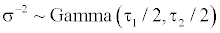. (20)

The precision parameter α of the DP prior can take a fixed value. Or, it can be treated as unknown and inferred from the analysis. Typically, a Gamma prior distribution is assumed to α and the statistical method for estimating α follows [20,21]. The computational implementation of the model is based on the marginalization of the DP and on the use of MCMC methods for conjugate priors [22-24]. Some details on posterior sampling of unknown parameters are provided in the Appendix.

A salient feature of the DPP model is that the distribution of uihas point masses located at previous draws uj ( j ≠ i ). This implies a clustering property: the values of u's (and hence θ's ) for the primary studies are clustered by the DPP model. For example, if ui represents a QTL location (relative to μ) in the i-th study, the clusters of u's on a specific chromosome can have meaningful implication for the number of putative QTLs and their locations. Let g1,..., gm be m distinct values among k estimates of QTL locations u1,...,uk , where 1≤m≤ k at the t-th iteration of the sampler. Then, the unique values of g1,..., gkinduce a partitioning into m clusters. For each cluster, say j, all the u's belonging to this cluster take on the same value gj . Biologically, each cluster can represent a hypothetic (meta-) QTL. In the Bayesian context, the marginal posterior distribution of the number of clusters (i.e., number of QTLs) can be constructed by directly counting the number of distinct values of u's at each iteration of the sampler. Following , the g's can be simulated from their fully conditional distributions, which in turn improves the chain mixing.

Results

Meta-analysis of a candidate gene effect: A simulation study

In this simulation study, a candidate gene effect was estimated for 30 independent populations each with a varying effect size (Table 1). Briefly, the effects of this gene in the first 10 study populations were generated from N(0, 2) and those for the remaining study populations were generated from N(8, 3). This obviously creates a mixture distribution when simulated gene effects for the 30 primary studies are pooled (Figure 1). For each primary study population, the sample variance si2 of the gene effect was assumed to be known and generated from a gamma distribution (shape=1, scale=0.25).

Candidate gene effect
Study Mean Variance
1 2.9694 0.1319
2 -0.8076 0.5277
3 -3.1172 0.3557
4 2.4559 0.0579
5 4.6262 0.0352
6 1.4093 0.1737
7 0.6137 0.1140
8 -1.5614 0.2686
9 1.6295 0.0167
10 -0.4216 0.0108
11 13.2721 0.3186
12 10.4219 0.3432
13 7.0050 0.2510
14 6.2682 0.0062
15 8.0910 0.2885
16 4.6931 0.1483
17 9.4562 0.2221
18 10.8908 0.0855
19 8.4665 0.0145
20 7.4688 0.0282
21 6.8679 1.1789
22 8.6690 0.4515
23 12.5752 0.0505
24 6.5805 0.0104
25 10.5907 0.0051
26 2.4470 0.2859
27 5.3087 0.2550
28 7.4948 0.1571
29 3.7154 0.1869
30 4.1030 0.1366

Table 1: Mean and sample variance of a candidate gene in 30 simulation populations.

Figure 1: Kernel density plots (bandwidth=1.25) of simulated effects of a candidate gene in 30 primary study populations: (a) populations whose candidate gene affects were generated from N(2,0); (b) populations whose candidate gene effects were generated from N(8,3); (b) mixtures of populations from both groups.

The R package “metaphor” was used to compute the parametric models . The overall mean of the candidate gene effects was estimated using equation (3) for the fixed-effect model and equation (10) for the random-effect model. Based on the meta-analytic fixedeffect model, the mean (standard error) of the candidate gene effect across the 30 populations was 6.35 (0.03), with a 95% confidential interval (CI) between 6.23 and 6.42. This overall gene effect was significant from zero (p<0.0001), and the test for heterogeneity was very significant as well: Q (df = 29) = 6709.69 with p < 0.0001. By taking the significant heterogeneity of the candidate gene effects into consideration, the random-effect model estimated the overall gene effect to be 5.41 with a standard error of 0.78. This estimate was closer to the weighted average of the simulated mean effects (5.33 = 0×33.33% + 8×66.67%) than that obtained from the fixed-effect model. The total amount of heterogeneity was estimated to be 18.05 with the standard error being 4.79. Furthermore, a Bayesian model under the heterogeneity assumption about the gene effects was implemented using an R programs that we developed, with unknown parameters estimated from posterior samples generated by posterior distributions (15), (16) and (17), respectively. Assuming a normal prior distribution for study-specific gene effects, the Bayesian analysis estimated the overall candidate gene effect to be 5.40, which was very close to the estimate obtained from the random-effect model.

Obviously, while this candidate had varied effects on the 30 study populations, knowing only the overall mean of the gene effects did not convey as much information as needed to postulate its influence on the trait. Forest plots  clearly show the relative strength (effect size) of this candidate gene in each of the primary studies, as obtained from the parametric random-effects model (Figure 2). Estimated effect size for these studies (each represented by a rectangle) and their CIs (each represented by a horizontal line) are plotted on the right-hand side of this graph, with the names of the studies listed on the left-hand side. The area of each rectangle is proportional to the study’s weight in the meta-analysis. The common effect size estimated by the meta-analysis is plotted as a diamond. A vertical line representing no effect is also shown. If the CI for an individual study overlaps with this line, the effect size for the individual study is not significant. The same interpretation applies to the overall mean of the candidate gene effect sizes estimated by the meta-analysis. Clearly, estimated candidate gene effects in the 30 individual studies varied dramatically, which made it hard to draw a consistent conclusion about this gene effect. Nevertheless, the meta-analytic random effect model estimated the 95% CI of the overall gene effect to be between 88.66 and 120.83. This is indication that this candidate gene effect is significant because its 95% CI does not overlap with the vertical line representing no effect. As a comparison, the posterior distribution of the overall gene effects, obtained from the Bayesian model, is shown below the forest plots (Figure 2).

Figure 2: Forest plot showing the candidate gene effects obtained from 30 primary study populations (rectangles) and the overall mean of the gene effect estimated from the meta-analytic random-effect analysis. Below the forest plot is the posterior distribution of the overall mean of the candidate gene effects estimated based on a Bayesian model under the heterogeneity model.

The DPpackage  was used to compute the Bayesian DPP model. The Markov chain Monte Carlo sampling consisted of 200,000 iterations, thinned at every one-tenth, with a burn-in period of 10,000 iterations. The saved samples are used for posterior inference. In this analysis, the parameter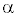was assumed to known and arbitrarily set to be 0.05. The number of clusters was estimated to be 8.70, with a 95% HPD interval between 8 and 10 clusters. The posterior mean (standard) of the overall mean of the gene effect was estimated to be 5.42 (0.74), which corresponded well to the estimate from the random-effect model. The posterior distributions of the cluster number, and the overall mean and variance of this candidate effects are shown in (Figure 3).

Figure 3: Posterior distributions of unknown parameters estimated from the non-parametric meta-analysis model with Dirichlet process prior: (a) clusters of gene effects among the 30 primary study populations; (b) mean of the baseline distribution, (c) variance of the baseline distribution.

By simulation, the candidate gene effects in the 30 populations actually represent a mixture of two normal distributions, N(0,2) and N(8,3). In reality, however, we do not know how many categories the mixture is actually formed with. This could cause some difficulties to the parametric mixture model because the exact number of components would need to be inferred. In contrast, the DPP model handles naturally a mixture with an unknown number of components. Our results show that the number of clusters of gene effects was estimated larger than that of simulated categories. This was because we arbitrarily assigned a small value to the parameter α. In the DPP model, the inference about the cluster number is sensitive to this parameter . Recall that α in the DPP model represents the degree of belief in G0 (which is assumed to be normal in the present analysis). In other words, α is interpretable as a measure of how close G0 is to the true but unknown distribution G. As α→ ∞ , the prior for G “concentrates” on G0. On the other hand, a small value of α indicates that the data is diffuse, and that the non-parametric model using the Dirichlet process prior would fit the data better than the parametric methods assuming a normal distribution of θ 's . This was exactly the case with the present simulation study. Although estimated number of clusters of gene effects (which is 8.79) was larger than the actual number of categories in the simulation (which is 2), the gene effect estimated for each of these study populations based on the DPP model corresponded very well to its simulated value (Figure 4). This result indicates that the DPP model tends to group populations with similar gene effects and hence fits the data very well, even if the inferred and the true numbers of categories may not necessarily match each other.

Figure 4: Estimation of the candidate gene effects using the non-parametric meta-analysis model with a Dirichlet process prior (DPP model): (a) correlation between simulated candidate gene effects from 30 primary study populations and their predicted value from the DPP model; (b) kernel density plots (bandwidth=0.3) of predicted candidate gene effects from the DPP model.

Meta-analysis of ADG QTL locations in swine chromosome 1

As an illustrative application, meta-analyses were conducted to combine QTL results on average daily gain (ADG) on swine chromosome 1 (SSC1). The summary data included means and 95% CIs of QTL position data extracted from the Animal QTLdb database (http://www.animalgenome.org/cgi-bin/QTLdb/index). This data set represented 21 ADG QTLs reported by 13 independent studies (Table 2). Estimated QTL position (POS) were obtained from the original studies and the corresponding standard errors (SE) were computed based on the 95% CI of QTL positions. For the QTL locations lacking reported CI (whose QTLID are marked by “*”), we assigned an average SE to each of them, for the sake of simplicity.

REFID QTLID CHR POS SE TRAIT SIGLEV
ISU0007 841 1 43.73 1.51 ADG ++
16978171 2846 1 101 4.52 ADG ++
16978171 2847 1 115 4.52 ADG ++
16415521 *2885 1 119.5 5.52 ADG NA
16415521 *2886 1 67.6 5.52 ADG NA
16478944 3133 1 143 6.51 ADG ++
12807782 3665 1 133.8 3.42 ADG ++
12807782 3673 1 60 3.91 ADG ++
17121599 3914 1 86.1 17.22 ADG ++
17121599 3917 1 105.8 17.22 ADG ++
9922390 448 1 139 5.36 ADG ++
9922390 447 1 139 5.36 ADG ++
10656927 139 1 79 0.18 ADG ++
10656927 140 1 79 0.19 ADG ++
11403749 170 1 140.5 2.42 ADG ++
12081807 659 1 140.5 8.80 ADG ++
ISU0002 319 1 12 1.86 ADG +
ISU0002 *320 1 121.3 5.52 ADG NA
9922390 446 1 139 5.36 ADG +
9263050 *495 1 102.9 5.52 ADG NA
11048919 *549 1 134 5.52 ADG NA

Table 2: Estimated positions and standard errors for average daily body weight gain (ADG) QTLs on swine chromosome 1 (data extracted from Animal QTLdb) 1

Tree plots of the 21 ADG QTL locations evidently indicated that they could represent several distinct ADG QTLs on SSC1 (Figure 5). Thus, estimating only a common (central) QTL location for these QTLs conveys no biological meaning. This renders that a meta-analytic fixedeffects model under the homogeneity assumption would not be a valid solution to this problem. Also, a random-effects model did not fit this situation well because the kernel density of the reported ADG QTL locations is clearly multi-modal (Figure 6a) and it by no means looks like a normal distribution.

Figure 5: Meta analysis of average daily body weight gain (ADG) QTL locations on swine chromosome 1: (a) Forest plots of reported ADG QTL locations obtained from 21 primary studies; (b) Posterior distribution of the overall mean of QTL locations on swine chromosome 1, obtained based on a Bayesian model under the heterogeneity assumption; (c) Plots of estimated ADG QTLs sorted by their respective locations.

Figure 6: Average daily body weight gain (ADG) QTL locations on swine chromosome 1: (a) Kernel density plot (bandwidth=5) of ADG QTL locations obtained from 21 primary studies; (b) Correlation between reported ADG QTL locations from 21 primary studies and the estimated QTL locations obtained using the non-parametric meta-analysis model with a Dirichlet process prior (DPP); (c) Posterior distribution of clusters of QTL locations inferred using the DPP model.

Instead, the DPP model can be used to analyze this dataset because it makes a weaker assumption about the form of study-specific QTL locations. A noting feature with the DPP model is that it introduces a clustering property, and each cluster can represent a distinct (meta-) QTLs. Based on the DPP model, estimated QTL locations corresponded very well to the reported QTL locations (Figure 6b). Plots of the posterior samples of the ADG QTL locations on swine chromosome 1 (Figure 6c) captured well the shape of the kernel density plot of the 21 reported ADG QTL locations (Figure 6b). This coincidence demonstrates that the DPP model could fit the data well when the distribution of QTL location (or any outcome) deviates from a normal distribution.

In this analysis, the parameter α was treated as an unknown parameter and inferred from its conditional posterior distribution. Assuming a beta prior distribution, α ~beta(2,20), the posterior mean (standard error) of α was estimated to be 0.335 (0.319). The number of clusters for the ADG QTL locations was estimated to be 6.73 with 95% HDP interval being between 6 and 8, indicating that the 21 reported ADG QTLs could present from 6 to 8 distinct ADG QTLs on SSC1. The mean (variance) for the baseline distribution were 76.99 (460.8), which corresponded to the overall estimate of QTL locations that could be obtained from parametric models. However, the interpretation of this overall mean in the baseline population is interpreted differently. The posterior distributions of the cluster number, and the mean and variance of the baseline distribution are shown in (Figure 7).

Figure 7: Posterior distributions of the cluster number (left), and the mean (middle) and variance (right) of the baseline distribution, estimated using the non-parametric meta-analysis model with a Dirichlet process prior. All results pertain to average daily body weight gain QTLs on swine chromosome 1.

Discussion and Conclusions

We have demonstrated that meta-analysis is a useful method for integration of information from multiple candidate gene studies and quantitative trait loci (QTL) mapping experiments. While individual studies may yield varied pictures of the candidate gene or QTL, pooling of results from several studies can reach a conclusion that is more consistent and stronger relative to individual studies. The use of parametric meta-analytic models to meta analysis of quantitative trait association and mapping studies is limited by their strong assumptions, such as the homogeneity or the normality assumption about studyspecific outcomes. These assumptions may not be valid in real situations. Instead, a non-parametric model with a Dirichlet process prior (DPP) makes weaker assumptions about study outcomes and it could fit data better than parametric models.

The DPP model involves a parametric family G0 (t) , a positive number α, and a prior distribution p(t) on t. Here., t is a set of parameters for G0. For example, t ={μ,σ2} if a normal baseline distribution is assumed. The process can be understood in two steps. In the first, parameters t are picked from p(t), and, in the second, G is picked from the Dirichlet distribution with parameters α andG0 (t) . This leads to the integral ∫DP (αG0 ) p(θ)dθ . Suppose we generate samples from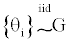. Because G is discrete, with positive probability, there will be ties among the θi's . In other words, the θi's will form clumps. When α is small, the first few probabilities ( Pj's ) add up to nearly 1, resulting in higher probability ties. This gives rise to important consequences regarding the posterior distribution of θ given the data γ. Consider the distribution of θi and let θ-i be θ without θi . Because of the propensity for clumping, the posterior of θi is more affected by those in θ-i whose values are close to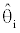. This property results in a way of pooling information that involves weighing results of similar studies more heavily .

Because the posterior distribution of the outcome parameter is discrete in the DPP model, it implies a clustering property that may have important biological implications. For example, inferred clusters of QTL locations could correspond to distinct QTL. With this clustering property, the DPP model could better capture the underlying patterns of the data variation than parametric models, thus providing an effective method for aggregating and synthesizing information from multiple independent studies.

Finally, the meta-analytic models are described without any studylevel covariate (also referred to as a moderator), yet it is possible to having such variables in the model as well. From a frequentist viewpoint, for example, with one or more moderator variables added to a fixed-effects model, it yields a meta-analytic fixed-effects-withmoderators model: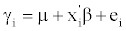(21)

where β is a vector containing all study-level covariates, and x'i is a row incidence vector (which takes values of 0 and 1, respectively). If one or more moderator variables are included in the random-effects model, it produces a meta-analytic mixed-effects-with-moderators model: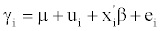(22)

where ui ~ N (0,σ2 ) . In the above model, the value of σ2 represents the “amount of residual heterogeneity” in the true effects or outcomes, that is, the amount of variability among the true effects or outcomes that is not accounted for by the moderators included in the model. With the presence of moderators, the models can be implemented similarly, yet requiring some necessary modifications in the algorithms.

Acknowledgement

This research is supported by the Wisconsin Agriculture Experiment Station and by grant NSF DMS-044371.

References

Select your language of interest to view the total content in your interested language

Article Usage

• Total views: 12303
• [From(publication date):
specialissue-2013 - Jun 20, 2019]
• Breakdown by view type
• HTML page views : 8459Can't read the image? click here to refresh